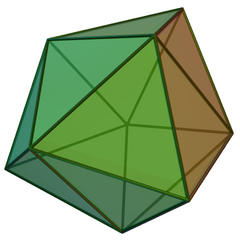A# deltahedronThe triaugmented triangular prism, a convex deltahedron.

A deltahedron is a polyhedron whose faces consist of equilateral triangles that are all the same size. Although there are an infinite number of different deltahedra, only eight of them are convex, as O. Rausenberger first showed in 1915. Among this group of eight, faces made of coplanar equilateral triangles sharing an edge (such as the rhombic dodecahedron) aren't allowed. The eight convex deltahedra have 4, 6, 8, 10, 12, 14, 16, and 20 faces.